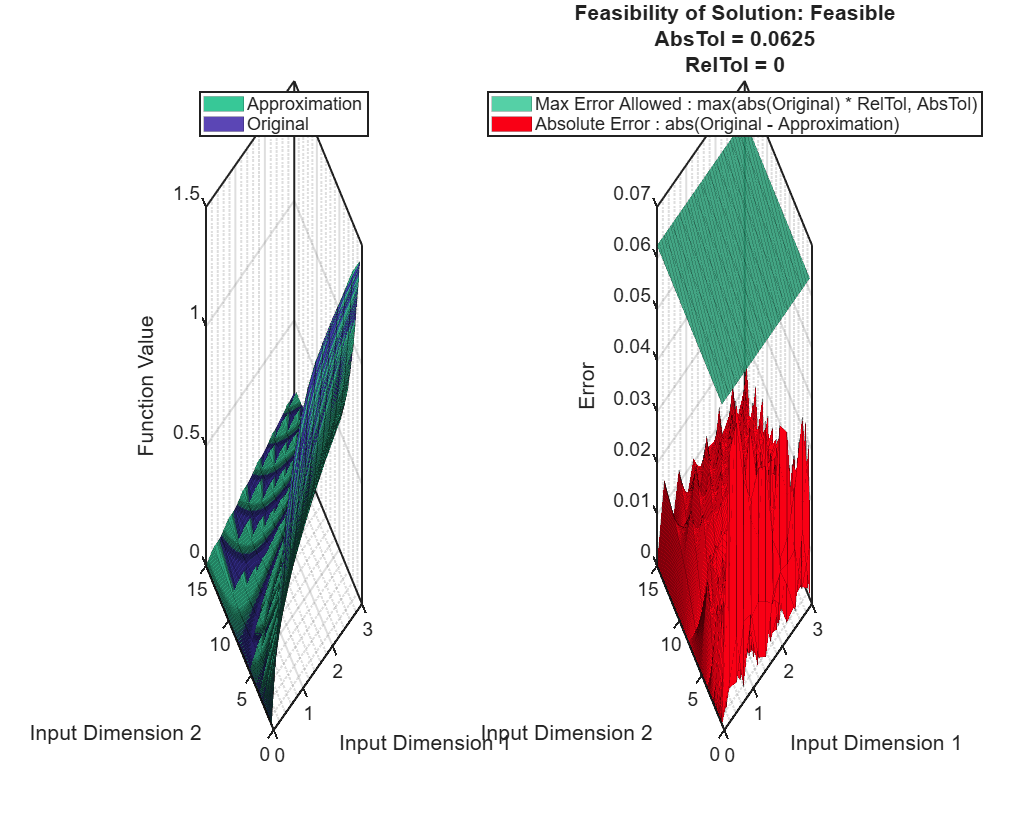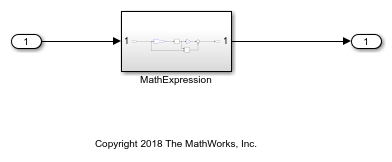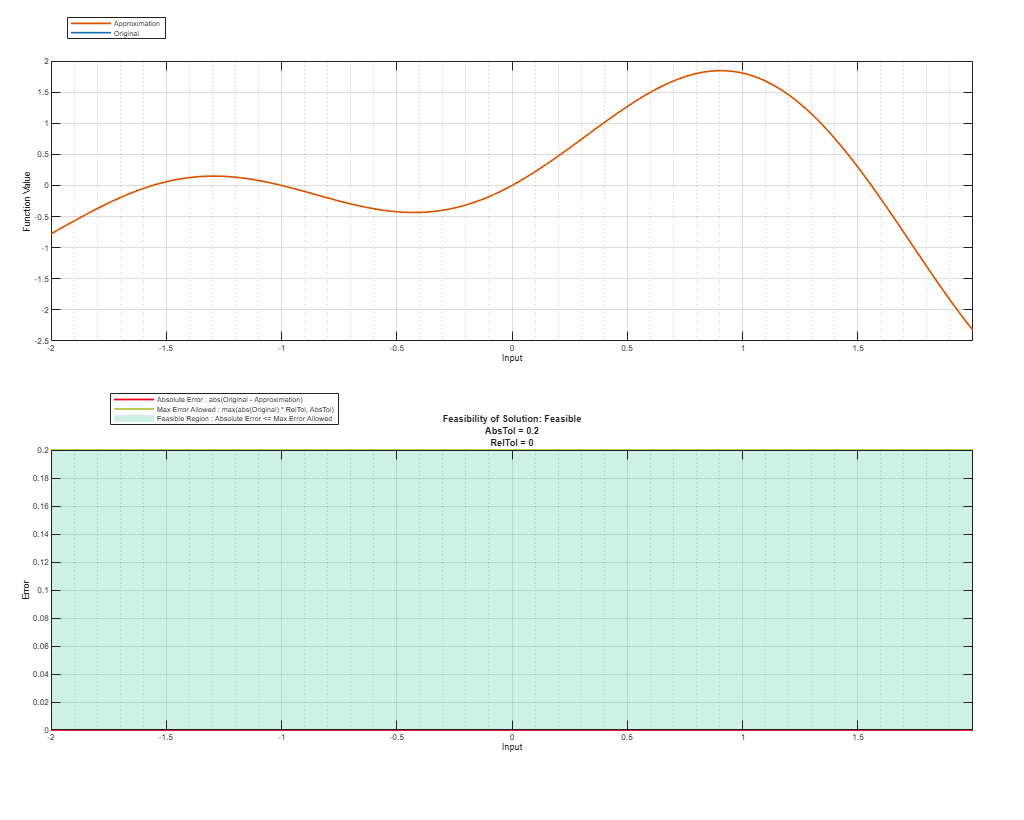Approximate Functions with a Direct Lookup Table

Using the Lookup Table Optimizer, you can generate a direct lookup table approximating a Simulink® block, or a function. Direct lookup tables are efficient to implement on hardware because they do not require any calculations.

Generate a Two-Dimensional Direct Lookup Table Approximation

Create a FunctionApproximation.Problem object specifying the function for which to generate the approximate. To generate a direct lookup table, set the interpolation method to None in the FunctionApproximation.Options object.

problem = FunctionApproximation.Problem('atan2');
problem.InputTypes = [numerictype(0,4,2) numerictype(0,8,4)];
problem.OutputType = fixdt(0,8,7);
problem.Options.Interpolation = "None";
problem.Options.AbsTol = 2^-4;
problem.Options.RelTol = 0;
problem.Options.WordLengths = 1:8;

Use the solve method to generate the optimal lookup table.

solution = solve(problem)
|  ID |  Memory (bits) | Feasible |   Table Size | Intermediate WLs | TableData WL |             Error(Max,Current) |
|   0 |          32768 |        1 |     [16 256] |            [4 8] |            8 |     6.250000e-02, 3.902460e-03 |
|   1 |          28672 |        1 |     [16 256] |            [4 8] |            7 |     6.250000e-02, 7.811287e-03 |
|   2 |          24576 |        1 |     [16 256] |            [4 8] |            6 |     6.250000e-02, 1.561990e-02 |
|   3 |          16384 |        1 |     [16 128] |            [4 7] |            8 |     6.250000e-02, 6.242016e-02 |
|   4 |          14336 |        1 |     [16 128] |            [4 7] |            7 |     6.250000e-02, 5.707978e-02 |
|   5 |          12288 |        1 |     [16 128] |            [4 7] |            6 |     6.250000e-02, 5.870371e-02 |
|   6 |          10240 |        0 |     [16 128] |            [4 7] |            5 |     6.250000e-02, 8.585766e-02 |
|   7 |           8192 |        0 |     [16 128] |            [4 7] |            4 |     6.250000e-02, 1.020576e-01 |

Best Solution
|  ID |  Memory (bits) | Feasible |   Table Size | Intermediate WLs | TableData WL |             Error(Max,Current) |
|   5 |          12288 |        1 |     [16 128] |            [4 7] |            6 |     6.250000e-02, 5.870371e-02 |

solution =

1x1 FunctionApproximation.LUTSolution with properties:

ID: 5
Feasible: "true"

Use the compare method to compare the output of the original function and the approximate.

compare(solution);Use the approximate method to generate a Simulink™ subsystem containing the generated direct lookup table.

approximate(solution)Generate a Direct Lookup Table Approximation for a Subsystem

This example shows how to approximate a Simulink™ subsystem with a direct lookup table.

Open the model containing the subsystem to approximate.

functionToApproximate = 'ex_direct_approximation/MathExpression';
open_system('ex_direct_approximation');To generate a direct lookup table, set the interpolation method to None.

problem = FunctionApproximation.Problem(functionToApproximate);
problem.Options.Interpolation = 'None';
problem.Options.RelTol = 0;
problem.Options.AbsTol = 0.2;
problem.Options.WordLengths = [7 8 9 16];
solution = solve(problem);
|  ID |  Memory (bits) | Feasible |   Table Size | Intermediate WLs | TableData WL |             Error(Max,Current) |
|   0 |        2097152 |        1 |        65536 |               16 |           32 |     2.000000e-01, 0.000000e+00 |
|   1 |            896 |        0 |          128 |                7 |            7 |     2.000000e-01, 2.265625e+00 |
|   2 |           1024 |        0 |          128 |                7 |            8 |     2.000000e-01, 2.265625e+00 |
|   3 |           1152 |        0 |          128 |                7 |            9 |     2.000000e-01, 2.265625e+00 |
|   4 |           2048 |        0 |          128 |                7 |           16 |     2.000000e-01, 2.267090e+00 |

Best Solution
|  ID |  Memory (bits) | Feasible |   Table Size | Intermediate WLs | TableData WL |             Error(Max,Current) |
|   0 |        2097152 |        1 |        65536 |               16 |           32 |     2.000000e-01, 0.000000e+00 |

Compare the original subsystem behavior to the lookup table approximation.

compare(solution);Generate a new subsystem containing the lookup table approximation.

approximate(solution);Replace the original subsystem with the new subsystem containing the lookup table approximation.

replaceWithApproximate(solution);

You can revert your model back to its original state using the revertToOriginal function. This function places the original subsystem back into the model.

revertToOriginal(solution);

Fixed-Point Designer DocumentationGet trial now